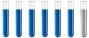## Molecular structure

Chemistry and homework help forum.

Organic Chemistry, Analytical Chemistry, Biochemistry, Physical Chemistry, Computational Chemistry, Theoretical Chemistry, High School Chemistry, Colledge Chemistry and University Chemistry Forum.

Share your chemistry ideas, discuss chemical problems, ask for help with scientific chemistry questions, inspire others by your chemistry vision!

Please feel free to start a scientific chemistry discussion here!

Discuss chemistry homework problems with experts!

Ask for help with chemical questions and help others with your chemistry knowledge!

Moderators: Xen, expert, ChenBeier

Dhamnekar Winod
Sr. Staff MemberPosts: 246
Joined: Sat Nov 21, 2020 10:14 am
Location: Mumbai[Bombay] and Mumbai Suburb,, Maharashtra State,India

### Molecular structure

What is the answer to the following question?

The potential energy of two charged particles at a certain distance can be found through the equation $$E= \frac{q_1q_2}{4\pi\epsilon_0 r}$$ where 1)r is a distance

2)
$$q_1$$ and $$q_2$$ are the charges

3)$$\epsilon_0= 8.8541878e-12F/m$$

Using the above equation, I computed the potential energy of four electrons located at the apices of a tetrahedron at a distance of 2.0 Å = 6.92e-18 Joules.

I computed the potential energy of four electrons located at the four corners of a square with edges of 2.0 Å =4.61e-18 Joules.

As four electrons located at the four corners of a square have lower potential energy than the potential energy of a tetrahedron, itis more stable.MOST12.png (41.41 KiB) Viewed 15089 times
Any science consists of the following process. 1) See 2)Hear 3)Smell,if needed 4)Taste, if needed 5)Think 6)Understand 7)Inference 8)Take decision [Believe or disbelieve, useful or useless, true or false, cause or effect, any other criteria]